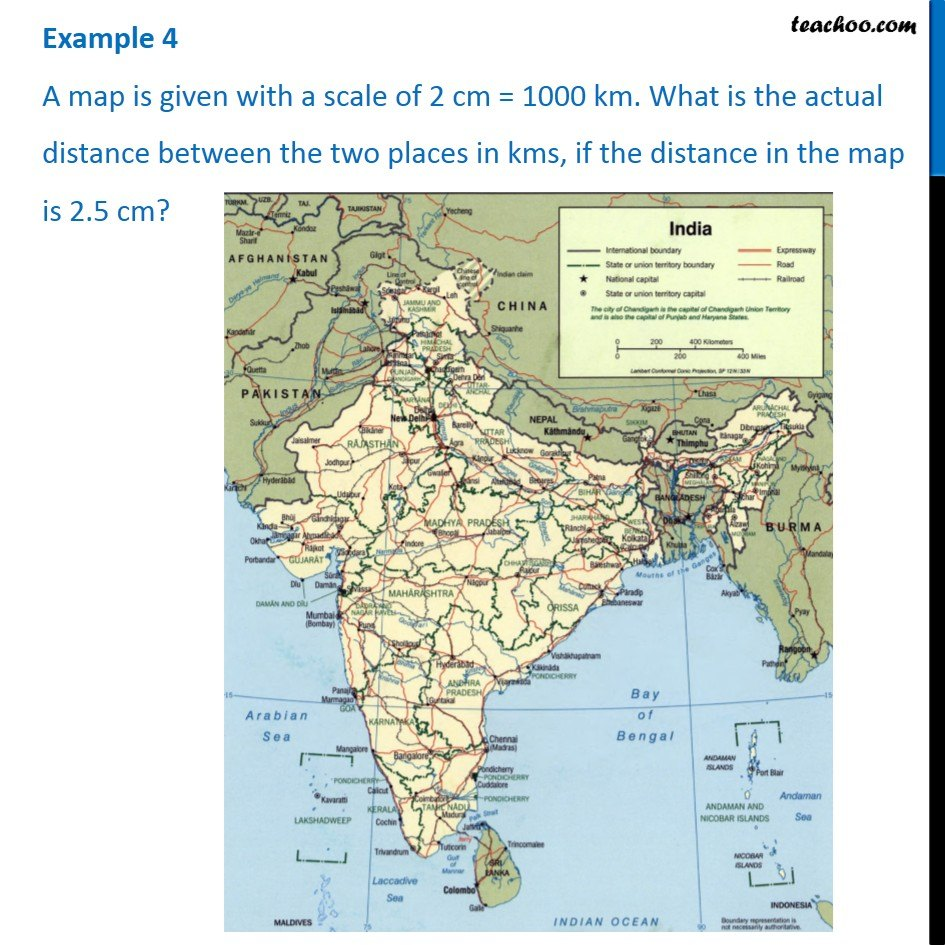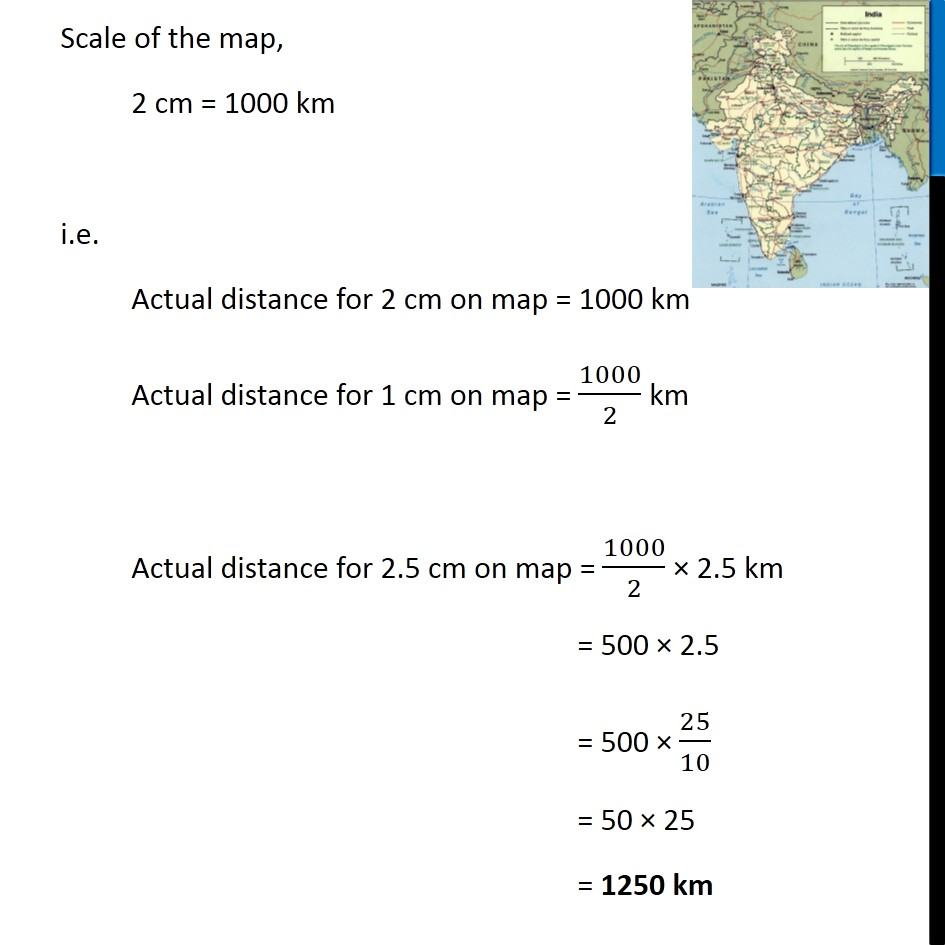Unitary Method

Chapter 8 Class 7 Comparing Quantities
Concept wiseIntroducing your new favourite teacher - Teachoo Black, at only ₹83 per month

### Transcript

Example 4 A map is given with a scale of 2 cm = 1000 km. What is the actual distance between the two places in kms, if the distance in the map is 2.5 cm? Scale of the map, 2 cm = 1000 km i.e. Actual distance for 2 cm on map = 1000 km Actual distance for 1 cm on map = 1000/2 km Actual distance for 2.5 cm on map = 1000/2 × 2.5 km = 500 × 2.5 = 500 × 25/10 = 50 × 25 = 1250 km